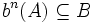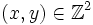# Sanov subgroup in SL(2,Z) is free of rank two

## Statement

Consider$G = SL(2,\mathbb{Z})$, the Special linear group:SL(2,Z) (?) (this is the subgroup of the general linear group:GL(2,Z) comprising the matrices of determinant one). Then, the subgroup of$G$ generated by the elements:$\begin{pmatrix}1 & 2 \\ 0 & 1\end{pmatrix}, \begin{pmatrix}1 & 0 \\ 2 & 1\end{pmatrix}$

is a free group with these two elements forming a freely generating set.

## Facts used

1. Ping-pong lemma (the form involving two elements): If$G$ acts on a set$X$, and$a,b \in G$ and$A,B \subseteq X$ are such that neither$A$ nor$B$ is contained in the other. Further, suppose that$b^n(A) \subseteq B$ and$a^n(B) \subseteq A$ for all nonzero integers$n$. Then, the subgroup of$G$ generated by$a$ and$b$ is a free group with$\{ a,b \}$ as a freely generating set.

## Proof

We construct an action that satisfies the hypothesis for fact (1) (the ping-pong lemma).

Let$X = \mathbb{Z}^2$ with$G$ acting on it the usual way (by matrix multiplication with column vectors). Consider the subsets:$A = \{ (x,y) \mid |y| > |x| \}, B = \{ (x,y) \mid |x| > |y| \}$.

We now prove the conditions for the ping-pong lemma:

• Neither of$A$ and$B$ is contained in the other: In fact, it is cler from the definition that they are disjoint non-empty sets.
•$a^n(B) \subseteq A$ for$n \ne 0$: Suppose$(x,y) \in \mathbb{Z}^2$ such that$|x| > |y|$. Then$a^n((x,y)) = (x,2nx + y)$. Since$|x| > |y|$,$|2nx + y| \ge |2n||x| - |y| \ge 2|x| - |y| = |x| + (|x| - |y|) > |x|$ by assumption.
•$b^n(A) \subseteq B$ for$n \ne 0$: This is similar to the previous part.